# 2 2021-05-13

## 2.1 00:14

Questions: $$\pi_1(X)$$ can be defined for schemes.

• What are the higher homotopy groups of schemes?
• What do they measure?
• More fundamentally, what do higher homotopy groups of spheres measure about $${\mathsf{Top}}$$ at all??
• What are the Eilenberg-MacLane spaces in $${\mathsf{Sch}}$$?
• What are the Moore spaces in $${\mathsf{Sch}}$$?
• There should be an axiomatic characterization of these coming from \begin{align*}\[model categories\end{align*} ].
• What are the “spheres” for $${\mathsf{Sch}}$$?
• What are \begin{align*}\[homotopy spheres\end{align*} ] in $${\mathsf{Sch}}$$?
• What are \begin{align*}\[homology spheres\end{align*} ] in $${\mathsf{Sch}}$$?

# 3 2021-05-12

## 3.2 20:46

Source: Frobenius exact symmetric tensor categories - Pavel Etingof. IAS Geometric/modular representation theory seminar. https://www.youtube.com/watch?v=7L06K7SL5qw

• Looking at modular representations of finite groups.

• Irreducible representations: hard, but a lot is known.
• Indecomposable representations: very hard, very little is known. Hard
• See tensor ideal, \begin{align*}\[Krull-Schmidt theorem\end{align*} ]: decomposition into indecomposables is essentially unique.

• Can take split Grothendieck ring.

• Symmetric tensor category $$\mathsf{C}$$:

• $$k{\hbox{-}}$$linear, so enriched in $${\mathsf{Vect}}_{/k}$$ for $$k= \mkern 1.5mu\overline{\mkern-1.5muk\mkern-1.5mu}\mkern 1.5mu$$ (no assumption on characteristic) So morphisms are vector spaces and composition is bilinear.
• Abelian
• Artinian: objects have finite length and $$\dim_K \mathsf{C}(X, Y) < \infty$$
• Monoidal: $$(\otimes, \one)$$ satisfying associativity and the pentagon axiom
• Symmetric monoidal: a symmetric braiding $$X\otimes Y \xrightarrow{\tau_{XY}} Y\otimes X$$ such that $$\tau_{YX} \circ \tau_{XY} = \operatorname{id}$$.
• Rigid: existence of duals and morphisms $$X\to X {}^{ \check{} }$$ plus rigidity axioms
• Compatibility of additive/multiplicative structures: implied when $$\otimes$$ is bilinear on morphisms.
• $$\mathop{\mathrm{End}}_{\mathsf{C}}(\one) = k$$.
• Example: $${\mathsf{Rep}}_k(G)$$ the category of finite-dimensional representations of $$G$$. Take $$G=1$$ to recover $${\mathsf{Vect}}_{/k}$$.

• Such a category is tannakian if there exists a fiber functor: a symmetric tensor functor $$F: \mathsf{C} \to {\mathsf{Vect}}_{/k}$$.

• Preserves tensor structure (hexagon axiom), preserves braiding, is exact.
• Implies automatically faithful.
• Can take forgetful functor from representations to underlying vector space.
• Called “fiber” because for spaces and local systems, one can take a fiber at a point
• Deligne-Milne show this is unique.
• Can define scheme of tensor automorphisms, $$G = \underline{{\operatorname{Aut}}}^\otimes(F) \in {\mathsf{Grp}}{\mathsf{Sch}}_{/k}$$.
• For an (additive) rigid symmetric monoidal category $$\mathsf{C}_{/k}$$ with $$\mathop{\mathrm{End}}_{\mathsf{C}}(\one) = k$$, for any $$f\in \mathsf{C}(X, X)$$ we can define its trace $$\operatorname{Tr}(f) \in \mathop{\mathrm{End}}_{\mathsf{C}}(\one)$$: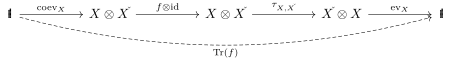• Can define a categorical dimension $$\dim X \in K$$ as $$\dim X\mathrel{\vcenter{:}}=\operatorname{Tr}(\operatorname{id}_X)$$.
• See theory of semisimplification.
• A morphism $$f\in \mathsf{C}(X, Y)$$ is negligible if for all $$g\in \mathsf{C}(Y, X)$$ we have $$\operatorname{Tr}(f\circ g) = 0$$.
• Negligible morphisms form a tensor ideal of morphisms: stable under composition and tensor product with other morphisms.
• Can form quotient $$\mkern 1.5mu\overline{\mkern-1.5mu\mathsf{C}\mkern-1.5mu}\mkern 1.5mu \mathsf{C} / \mathsf{N}$$: full subcategory where $$\mkern 1.5mu\overline{\mkern-1.5mu\mathsf{C}\mkern-1.5mu}\mkern 1.5mu(X, Y) \mathrel{\vcenter{:}}=\mathsf{C}(X, Y) / \mathsf{N}(X, y)$$, i.e. form the vector space quotient of the hom sets.
• Still monoidal.
• Generally nasty, but if trace of any nilpotent endomorphism in $$\mathsf{C}$$ (e.g. when $$\mathsf{C}$$ admits a monoidal functor to an abelian STC), then $$\mkern 1.5mu\overline{\mkern-1.5mu\mathsf{C}\mkern-1.5mu}\mkern 1.5mu$$ is a semisimple STC, and in particular is abelian and every object is a direct sum of simple objects.
• True if $$\mathsf{C}$$ is abelian: take any nilpotent endomorphism, filter by kernels of powers and take the associated graded. Trace of original equals trace of associated graded, but the latter is zero.
• Can compute trace after pushing through an abelian functor.
• $$\mkern 1.5mu\overline{\mkern-1.5mu\mathsf{C}\mkern-1.5mu}\mkern 1.5mu$$ is the semisimplification of $$\mathsf{C}$$
• simple objects in $$\mkern 1.5mu\overline{\mkern-1.5mu\mathsf{C}\mkern-1.5mu}\mkern 1.5mu$$ are indecomposables in $$\mathsf{C}$$ of nonzero dimension.
• Run into functors that aren’t exact on either side, e.g. the Frobenius.
• But still additive, and every additive functor on a semisimple category is exact.
• Frobenius functors: take $$X^{\otimes p}$$, allow cyclic permutations $$c$$ with $$c^p = 1$$, get equivariance with respect to $${\mathbb{Z}}/p$$.
• Thus $$X^{\otimes p} \in \mathsf{C} \boxprod {\mathsf{Rep}}_{/k} {\mathbb{Z}}/p$$, the Deligne tensor product.
• Can take $$\operatorname{id}_{\mathsf{C}} \boxprod \ss$$, i.e. semisimplification on the 2nd component, to get an additive monoidal twisted-linear functor $$\mathop{\mathrm{Fr}}: \mathsf{C} \to \mathsf{C} \boxprod \Ver_p$$
• Here $$\Ver_p$$ is a Verlinde category: just denotes $$\mkern 1.5mu\overline{\mkern-1.5mu\mathsf{C}\mkern-1.5mu}\mkern 1.5mu$$ with a modified tensor product formula…?
• Can filter $$X^{\otimes p}$$ by $$\mathop{\mathrm{Fr}}_i \mathrel{\vcenter{:}}=\ker (1-c)^i$$.

# 4 2021-05-11

## 4.1 12:48

What is an isocrystal?

What does it mean to be crystalline?

What is a symplectic basis?

What is the Mordell-Weil sieve?

How can one pass from p-adic solutions to rational solutions?

## 4.2 13:05

What is the conductor of an elliptic curve?

See Serre’s open image theorem.

## 4.3 13:11

What is inertia?

What is the cyclotomic character?

## 4.5 14:11

See Goursat’s lemma in group theory.

# 5 2021-05-10

## 5.1 12:34

We haven’t been able to classify the rational points on \begin{align*}\[modular curves\end{align*} ]!

## 5.2 13:16

Reference: Kirsten Wickelgren, Colloquium Presentation: zeta functions and a quadratic enrichment. Rational Points and Galois Representations workshop

See \begin{align*}\[motivic homotopy\end{align*} ].

• Dualizability?• Works in a symmetric monoidal category.
• Finite dimensionality is replaced by objects being \begin{align*}\[dualizable\end{align*} ], so for \begin{align*} \one & \xrightarrow{m} A\otimes B \\ B\otimes A & \xrightarrow{\varepsilon} \one ,\end{align*} require• See \begin{align*}\[Atiyah duality\end{align*} ]: define the dual of $$M$$ as $$M^{-\mathop{\mathrm{{T}}}M}$$, the \begin{align*}\[Thom space\end{align*} ] of (minus) the tangent bundle.

• Define the trace: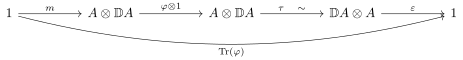• Then $$\operatorname{Tr}(\phi) \in \mathop{\mathrm{End}}_{\mathsf{C}}(\one, \one)$$ is an endomorphism of the unit.

• Example: Lefschetz fixed point theorem, \begin{align*} \operatorname{Tr}(\phi) = \sum_{x\in M, \phi(x) = x} \mathop{\mathrm{ind}}_x \phi \in \mathop{\mathrm{End}}_{{\mathsf{ho}}{\mathsf{Sp}}}(\one) \xrightarrow{\deg \,\, \sim} {\mathbb{Z}} ,\end{align*} where we take the degree of a map between spheres.

• $${\mathbb{S}}= \one \in {\mathsf{ho}}{\mathsf{Sp}}$$.

• Use that $$H^*({-}, {\mathbb{Q}})$$ preserves tensor products, and apply the Kunneth formula: \begin{align*} H^*(\operatorname{Tr}(\phi)) &= \operatorname{Tr}(H^*(\phi)) \\ \implies \sum (-1)^i \operatorname{Tr}( H^i(\phi); H^i(M) {\circlearrowleft}) &= \sum_{x\in M, \phi(x) = x} \mathop{\mathrm{ind}}_x \varphi .\end{align*}

• Rationality of $$\zeta$$:

• Use hocolims to glue spaces, but may not work in schemes.

• Example: take $$X \mathrel{\vcenter{:}}={\mathbb{P}}^n/{\mathbb{P}}^{n-1}$$, then we’d want $$X({\mathbb{C}}) \cong S^{2n}$$ and $$X({\mathbb{R}}) \cong S^n$$

• Problem: this quotient isn’t a \begin{align*}\[scheme\end{align*} ]. Can freely add these limits.

• We want $${\mathbb{P}}^i / {\mathbb{P}}^{i-1}$$ to be the building blocks or cells

• Morel and Voevodsky, $${\mathbb{A}}^1$$ \begin{align*}\[stable homotopy category\end{align*} ] over $$k$$, denoted $${\operatorname{SH}}(k)$$.

• Take an analog of degree, the Morel degree: \begin{align*} \deg: [{\mathbb{P}}^n/{\mathbb{P}}^{n-1}, {\mathbb{P}}^n/{\mathbb{P}}^{n-1} ] \xrightarrow{} {\operatorname{GW}}(k) .\end{align*}

• Recovers degree on $$X({\mathbb{C}})$$.
• \begin{align*}\[Grothendieck-Witt\end{align*} ] group: formal differences of isomorphism classes of nondegenerate symmetric bilinear forms.

• Allow orthogonal direct sum and orthogonal direct difference.
• Special form: the hyperbolic form \begin{align*} \left\langle{1}\right\rangle + \left\langle{-1}\right\rangle = \left\langle{a}\right\rangle + \left\langle{-a}\right\rangle = \begin{bmatrix} 0 & 1 \\ 1 & 0 \end{bmatrix} .\end{align*}

• See \begin{align*}\[rank\end{align*} ], \begin{align*}\[signature\end{align*} ], \begin{align*}\[discriminant\end{align*} ] of forms.

• Trace here will take values in $${\operatorname{GW}}(k)$$.

• Lefschetz fixed point theorem due to Hoyois:

• Notation: $$dZ^{{\mathbb{A}}^1}(t) = {\frac{\partial }{\partial t}\,} \log \zeta^{{\mathbb{A}}^1}(t) = \sum_{m\geq 1} \operatorname{Tr}(\phi^m)t^{m-1}$$.

• Prop: \begin{align*} \operatorname{rank}dZ^{{\mathbb{A}}^1}(t) = {\frac{\partial }{\partial t}\,} \log .\end{align*}

• See Kapranov motivic zeta functions:

• Define $$K_0({\mathsf{Var}}_k)$$ to be the group completion of varieties under cut-and-paste
• Define \begin{align*} Z_X^m(t) \mathrel{\vcenter{:}}=\sum_{m\geq 0} [\operatorname{Sym}^m X] t^m \in \mathop{\mathrm{{\mathsf{K} }}}_0 ({\mathsf{Var}}_k) {\left[\left[ t \right]\right] } .\end{align*}
• Define an \begin{align*}\[Euler characteristic\end{align*} ] \begin{align*} \chi_C^{{\mathbb{A}}^1}: K_0({\mathsf{Var}}_k) \to {\operatorname{GW}}(k) .\end{align*}
• See \begin{align*}\[Euler class\end{align*} ], \begin{align*}\[Hopf map\end{align*} ]

• Major point: this is genuinely something new, isn’t just recovered by taking the compactly supported euler characteristic:

• Defines a zeta function for any endomorphism of any variety. Doesn’t need to be over $${\mathbb{F}}_p$$, and doesn’t need to have Frobenius!

## 5.3 16:35

Reference: Foling Zou, Nonabelian Poincare duality theorem and equivariant factorization homology of Thom spectra. MIT Topology Seminar.

• Factorization homology setup: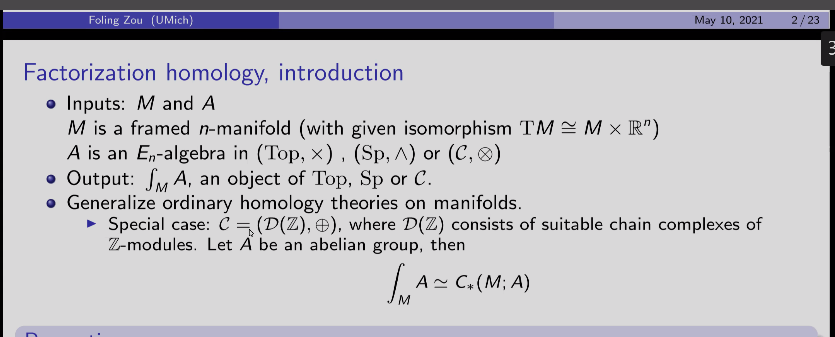• See \begin{align*}\[lambda sequences\end{align*} ], something like a functor $${\mathsf{FinSet}}^{\operatorname{op}}\to \mathsf{C}$$?

• See \begin{align*}\[Day convolution\end{align*} ] as an example of a monoidal product.

• Another example: the \begin{align*}\[Kelly product\end{align*} ]: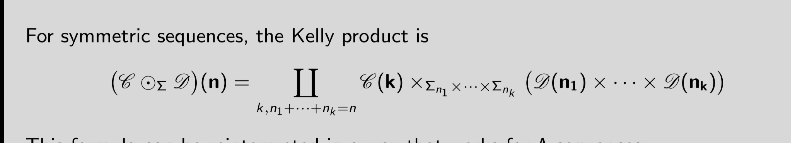• Can define operads and reduced operads as monoids in certain categories: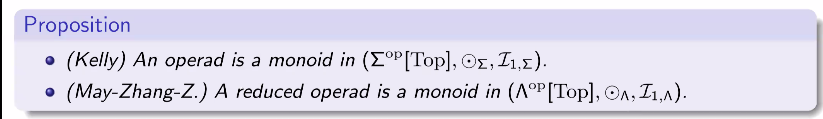• See \begin{align*}\[monadic bar construction\end{align*} ] and monoidal \begin{align*}\[bar construction\end{align*} ].

• Examples of factorization homology: \begin{align*} \int_{S^1}A &&\simeq{\operatorname{THH}}(A) \\ \int_{T^n}A &&\simeq{\operatorname{THH}}^n(A) && \text{iterated THH} .\end{align*}

• For $$\sigma$$ the \begin{align*}\[sign representation\end{align*} ], $$\int_{S^\sigma} A \simeq\operatorname{THR}(A)$$ for $$E_\sigma{\hbox{-}}C_2$$ spectra. See Horev, Hessolholt-Madsen.

• Axiomatic approach to factorization homology: take a left \begin{align*}\[Kan extension\end{align*} ] of the following: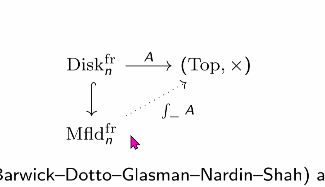• Theorem: equivariant nonabelian Poincaré duality: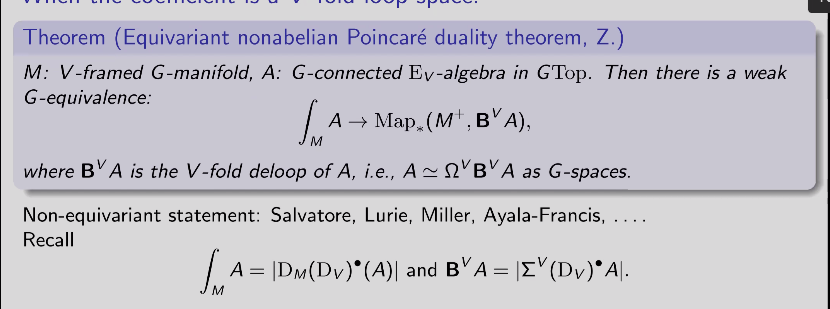• What is the \begin{align*}\[virtual dimension\end{align*} ] of a bundle?

• $${\operatorname{Pic}}$$: subcategory of invertible objects.

• \begin{align*}\[Thom spectrum\end{align*} ] functor:

where $$R{\hbox{-}}$$line is the $$\infty{\hbox{-}}$$category of line bundles up to equivalence?

• Preserves $$G{\hbox{-}}$$colimits, so formally the Thom spectrum functor commutes with factorization homology.

• In proof of theorem, use nonabelian Poincaré duality to reduce a complicated gadget to a mapping space.

• Also appears as a step in a later proof identifying $${\operatorname{THH}}_{C_2} (\mathop{\mathrm{{\mathsf{H}\mkern-3mu }}}{\mathbb{F}}_2) \approx \mathop{\mathrm{{\mathsf{H}\mkern-3mu }}}{\mathbb{F}}_2 \wedge(\mathop{\mathrm{{\Omega}}}S^3)_+$$.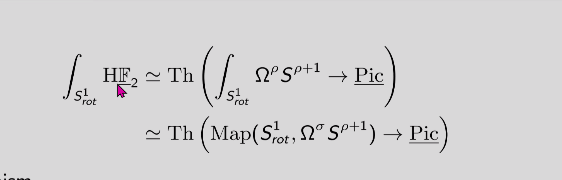• For $$\operatorname{THR}$$ on the algebra side, see Teena Gerhardt’s work? Haynes Miller suggests looking at the de Rham Witt complex.

# 6 2021-05-08

## 6.1 22:55

Not every simplicial complex is a PL manifold:

# 7 2021-05-06

## 7.1 11:15

Reference: Arpon Raksit - Hochschild homology and the derived de Rham complex revisited. https://www.youtube.com/watch?v=E84gVDm1kvM

• Algebraic de Rham complex: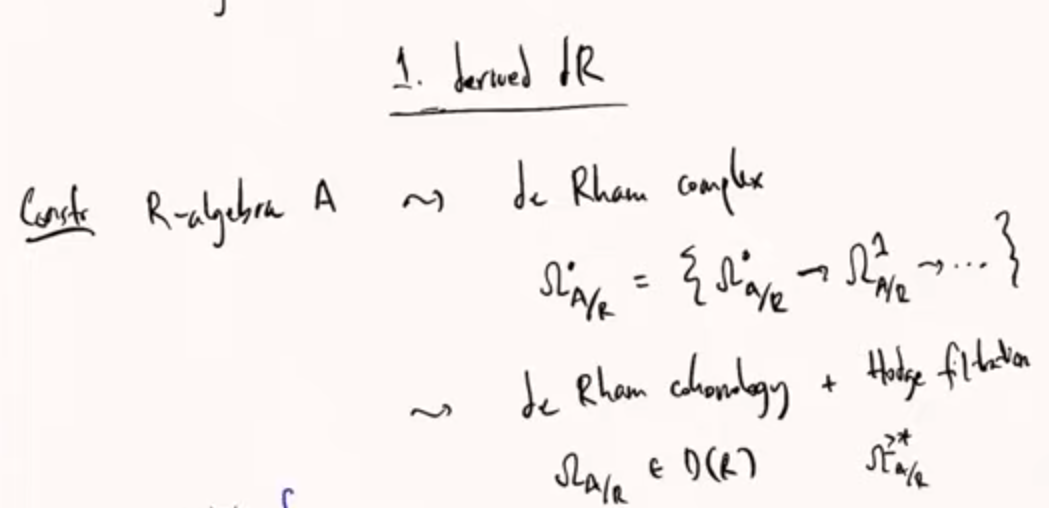• Can get a derived version: take non-abelian derived functors, i.e. take a simplicial resolution by simplicial polynomial algebras and apply the functor to the resolution.

• Define $$\mathop{\mathrm{{\mathbb{L} }}}\Omega^1 _{A/R}$$ to be the cotangent complex and take derived exterior powers for the other degrees.

• Derived Hodge filtration may not be complete, so take completion: take cone (cofiber?) in derived category and take a hocolim.